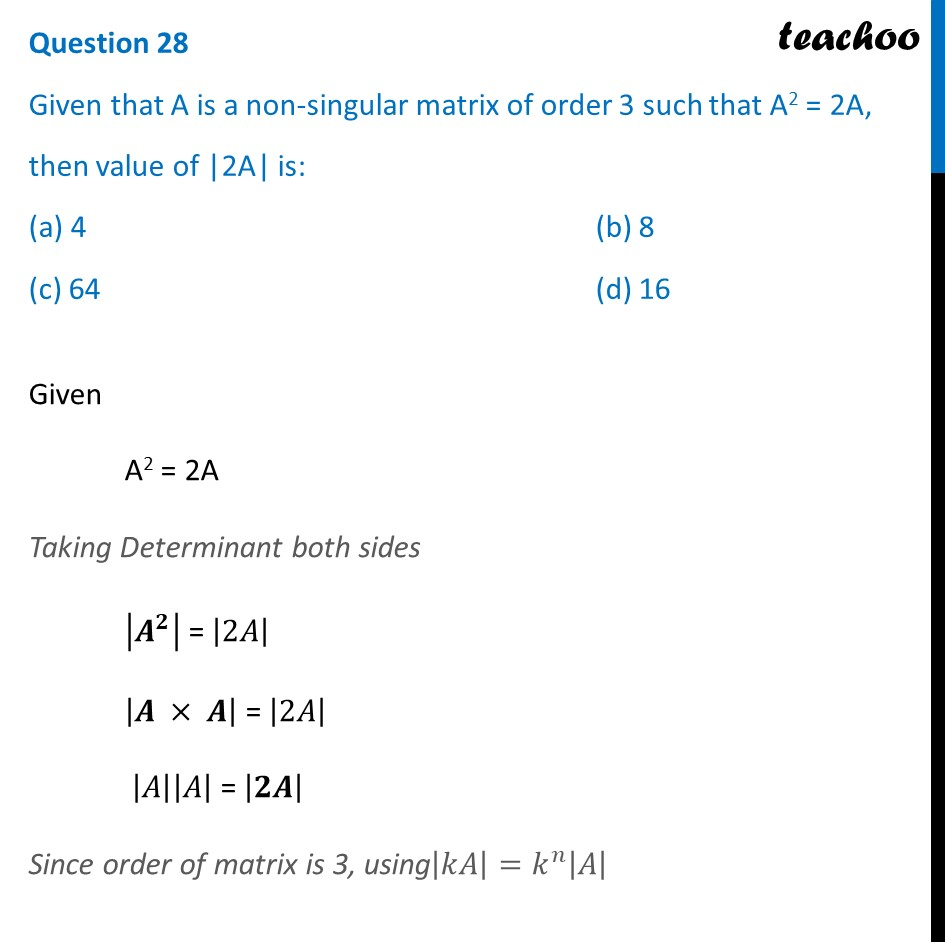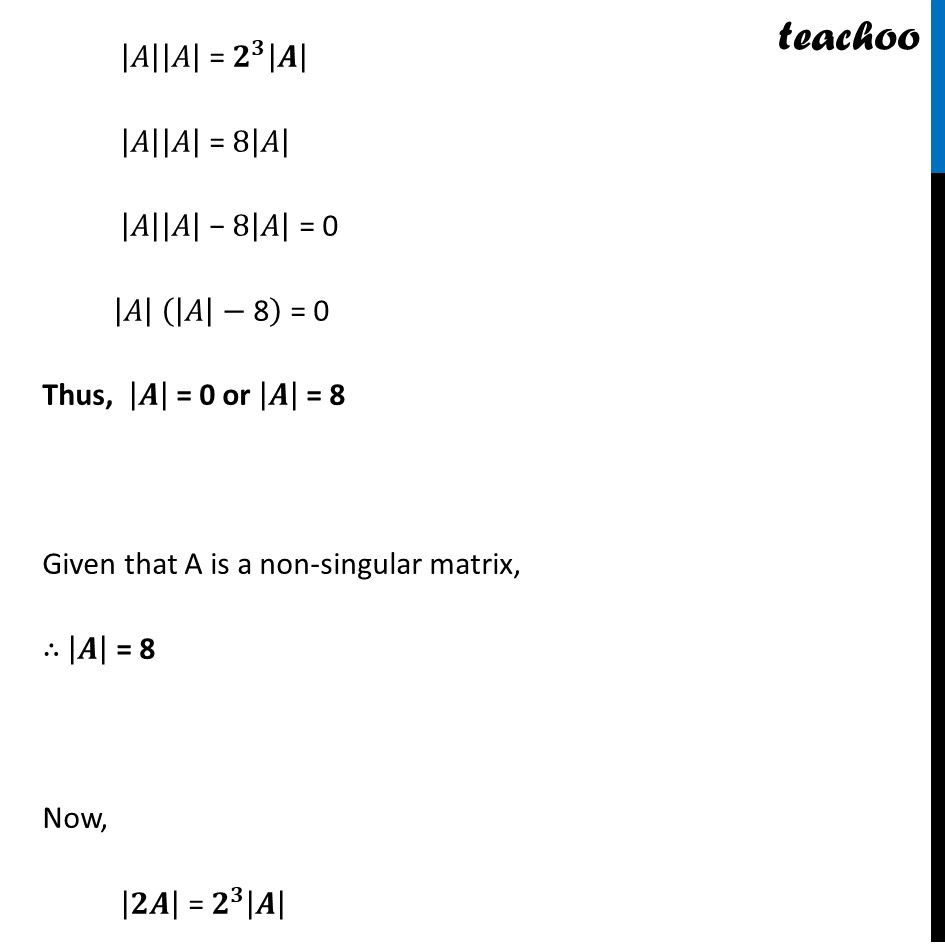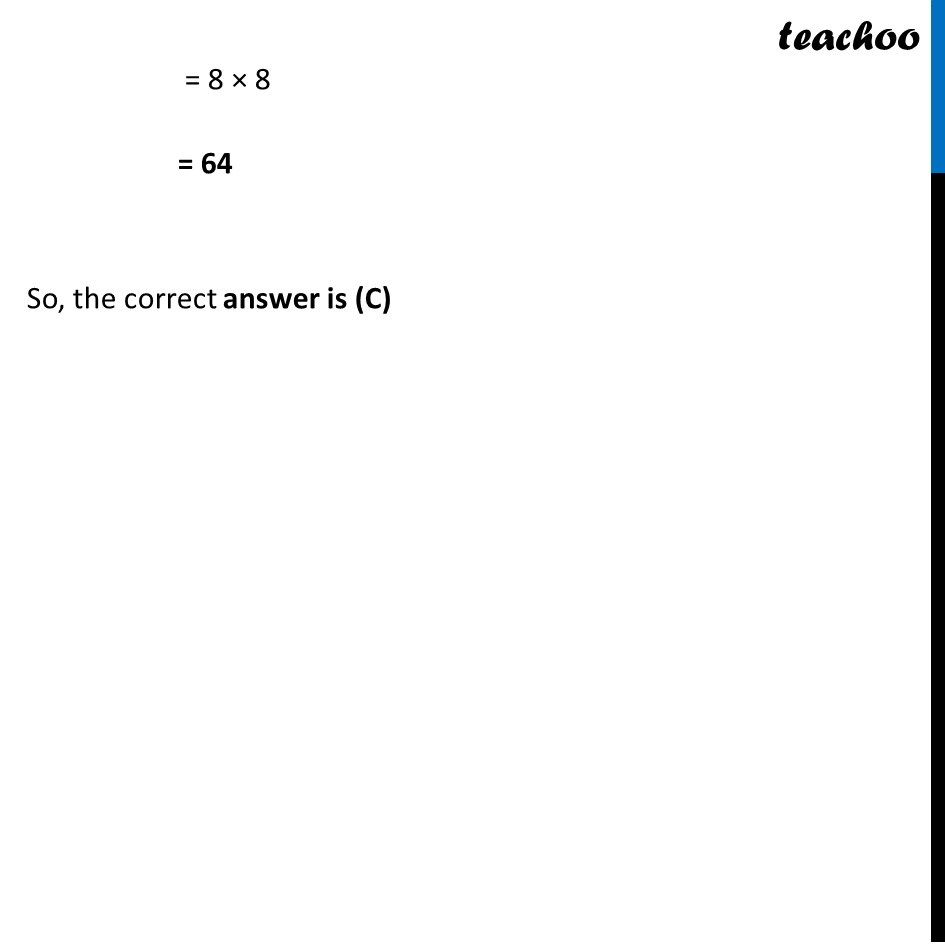CBSE Class 12 Sample Paper for 2022 Boards (MCQ Based - for Term 1)

Class 12
Solutions of Sample Papers and Past Year Papers - for Class 12 Boards

## (a) 4             (b) 8  (c) 64           (d) 16Get live Maths 1-on-1 Classs - Class 6 to 12

### Transcript

Question 28 Given that A is a non-singular matrix of order 3 such that A2 = 2A, then value of |2A| is: (a) 4 (b) 8 (c) 64 (d) 16 Given A2 = 2A Taking Determinant both sides |𝑨^𝟐 | = |2𝐴| |𝑨 × 𝑨| = |2𝐴| |𝐴||𝐴| = |𝟐𝑨| Since order of matrix is 3, using|𝑘𝐴|=𝑘^𝑛 |𝐴| |𝐴||𝐴| = 𝟐^𝟑 |𝑨| |𝐴||𝐴| = 8|𝐴| |𝐴||𝐴| − 8|𝐴| = 0 |𝐴| (|𝐴|−"8" ) = 0 Thus, |𝑨| = 0 or |𝑨| = 8 Given that A is a non-singular matrix, ∴ |𝑨| = 8 Now, |𝟐𝑨| = 𝟐^𝟑 |𝑨| = 8 × 8 = 64 So, the correct answer is (C)